# The Simpler Derivation of Logistic Regression

Logistic regression is one of the most popular ways to fit models for categorical data, especially for binary response data. It is the most important (and probably most used) member of a class of models called generalized linear models. Unlike linear regression, logistic regression can directly predict probabilities (values that are restricted to the (0,1) interval); furthermore, those probabilities are well-calibrated when compared to the probabilities predicted by some other classifiers, such as Naive Bayes. Logistic regression preserves the marginal probabilities of the training data. The coefficients of the model also provide some hint of the relative importance of each input variable.

While you don’t have to know how to derive logistic regression or how to implement it in order to use it, the details of its derivation give important insights into interpreting and troubleshooting the resulting models. Unfortunately, most derivations (like the ones in [Agresti, 1990] or [Hastie, et.al, 2009]) are too terse for easy comprehension. Here, we give a derivation that is less terse (and less general than Agresti’s), and we’ll take the time to point out some details and useful facts that sometimes get lost in the discussion.

To make the discussion easier, we will focus on the binary response case. We assume that the case of interest (or “true”) is coded to 1, and the alternative case (or “false”) is coded to 0.

The logistic regression model assumes that the log-odds of an observation y can be expressed as a linear function of the K input variables x:Here, we add the constant term b0, by setting x0 = 1. This gives us K+1 parameters. The left hand side of the above equation is called the logit of P (hence, the name logistic regression).

Let’s take the exponent of both sides of the logit equation.This immediately tells us that logistic models are multiplicative in their inputs (rather than additive, like a linear model), and it gives us a way to interpret the coefficients. The value exp(bj) tells us how the odds of the response being “true” increase (or decrease) as xj increases by one unit, all other things being equal. For example, suppose bj = 0.693. Then exp(bj) = 2. If xj is a numerical variable (say, age in years), then every year’s increase in age doubles the odds of the response being true — all other things being equal. If xj is a binary variable (say, sex, with female coded as 1 and male as 0), then if the subject is female, then the response is two times more likely to be true than if the subject is male, all other things being equal.

We can also invert the logit equation to get a new expression for P(x):The right hand side of the top equation is the sigmoid of z, which maps the real line to the interval (0, 1), and is approximately linear near the origin. A useful fact about P(z) is that the derivative P'(z) = P(z) (1 – P(z)). Here’s the derivation: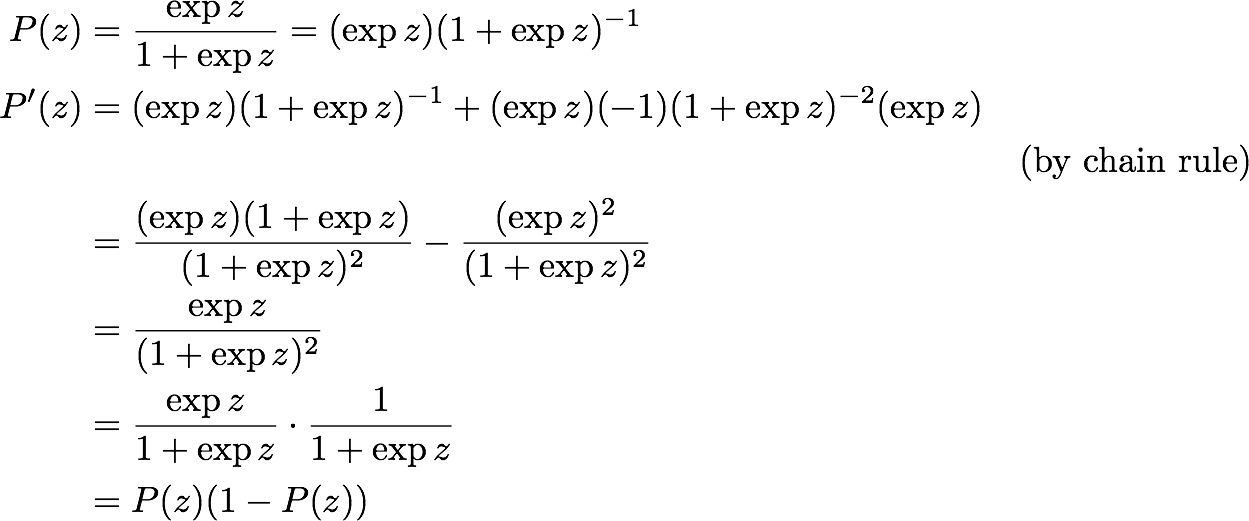Later, we will want to take the gradient of P with respect to the set of coefficients b, rather than z. In that case, P'(z) = P(z) (1 – P(z))z‘, where ‘ is the gradient taken with respect to b.

The solution to a Logistic Regression problem is the set of parameters b that maximizes the likelihood of the data, which is expressed as the product of the predicted probabilities of the N individual observations.(X, y) is the set of observations; X is a K+1 by N matrix of inputs, where each column corresponds to an observation, and the first row is 1; y is an N-dimensional vector of responses; and (xi, yi) are the individual observations.

It’s generally easier to work with the log of this expression, known (of course) as the log-likelihood.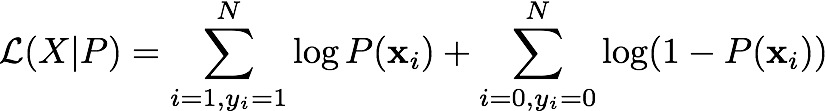Maximizing the log-likelihood will maximize the likelihood. As a side note, the quantity −2*log-likelihood is called the deviance of the model. It is analogous to the residual sum of squares (RSS) of a linear model. Ordinary least squares minimizes RSS; logistic regression minimizes deviance. A useful goodness-of-fit heuristic for a logistic regression model is to compare the deviance of the model with the so-called null deviance: the deviance of the constant model that returns only the global response probability for every data point. One minus the ratio of deviance to null deviance is sometimes called pseudo-R2, and is used the way one would use R2 to evaluate a linear model.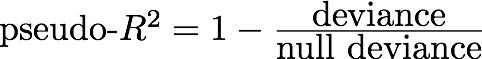Traditional derivations of Logistic Regression tend to start by substituting the logit function directly into the log-likelihood equations, and expanding from there. The derivation is much simpler if we don’t plug the logit function in immediately. To maximize the log-likelihood, we take its gradient with respect to b:where Pi is shorthand for P(xi). The maximum occurs where the gradient is zero.

We can expand this equation further, when we remember that P’ = P(1-P):The last line merges the two cases (yi = 1 and yi = 0) into a single sum. We can now cancel terms and set the gradient to zero. This gives us the set of simultaneous equations that are true at the optimum:Notice that the equations to be solved are in terms of the probabilities P (which are a function of b), not directly in terms of the coefficients b themselves. This means that logistic models are coordinate-free: for a given set of input variables, the probabilities returned by the model will be the same even if the variables are shifted, combined, or rescaled. Only the values of the coefficients will change.

The other thing to notice from the above equations is that the sum of probability mass across each coordinate of the xi vectors is equal to the count of observations with that coordinate value for which the response was true. For example, suppose the jth input variable is 1 if the subject is female, 0 if the subject is male. ThenIn other words, the summed probability mass for the female subjects equals the count of female subjects with the response “true”. It is also true that the sum of all the probability mass over the entire training set will equal the number of “true” responses in the training set. This is what we mean when we say that logistic regression preserves the marginal probabilities of the training data.

Solving for the Coefficients

The most straightforward way to solve for the coefficients b is Newton’s method. The Fisher scoring method that is used in most off-the-shelf implementations is a more general variation of Newton’s method; it works on the same principles. We will describe solving for the coefficients using Newton’s method.

Suppose you have a vector valued function f: y = f(b). You want to find the value bopt such that f(b)opt = 0. Assuming that we start with an initial guess b0, we can take the Taylor expansion of f around b0:Here, f‘ is a matrix; it is the Jacobean of first derivatives of f with respect to b. Setting the left hand side to zero, we can solve for Δ asWe then update our estimate for b: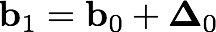and iterate until convergence.

In our case, f is the gradient of the log-likelihood, and its Jacobean is the Hessian (the matrix of second derivatives) of the log-likelihood function.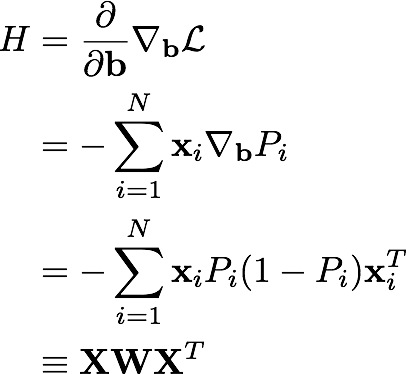where W is a diagonal matrix of the derivatives P’i, and the ith column of X corresponds to the ith observation. So we can solve for Δ at each iteration as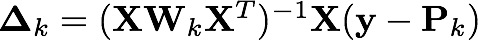where W is the current matrix of derivatives, y is the vector of observed responses, and Pk is the vector of probabilities as calculated by the current estimate of b.

Compare this to the solution of a linear regression: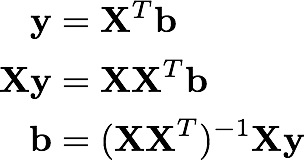Comparing the two, we can see that at each iteration, Δ is the solution of a weighted least square problem, where the “response” is the difference between the observed response and its current estimated probability of being true. This is why the technique for solving logistic regression problems is sometimes referred to as iteratively re-weighted least squares. Generally, the method does not take long to converge (about 6 or so iterations).

Thinking of logistic regression as a weighted least squares problem immediately tells you a few things that can go wrong, and how. For example, if some of the input variables are correlated, then the Hessian H will be ill-conditioned, or even singular. This will result in large error bars (or “loss of significance”) around the estimates of certain coefficients. It can also result in coefficients with excessively large magnitudes, and often the wrong sign. If an input perfectly predicts the response for some subset of the data (at no penalty on the rest of the data), then the term Pi (1 – Pi) will be driven to zero for that subset, which will drive the coefficient for that input to infinity (if the input perfectly predicted all the data, then the residual (yPk) has already gone to zero, which means that you are already at the optimum).

On the other hand, the least squares analogy also gives us the solution to these problems: regularized regression, such as lasso or ridge. Regularized regression penalizes excessively large coefficients, and keeps them bounded. If you are implementing your own logistic regression procedure, rather than using a package, then it is straightforward to implement a regularized least squares for the iteration step (as Win-Vector has done). But even if you are using an off-the-shelf implementation, the above discussion will help give you a sense of how to interpret the coefficients of your model, and how to recognize and troubleshoot some issues that might arise.

Conclusion

Here is what you should now know from going through the derivation of logistic regression step by step:

– Logistic regression models are multiplicative in their inputs.

– The exponent of each coefficient tells you how a unit change in that input variable affects the odds ratio of the response being true.

– Logistic regression is coordinate-free: translations, rotations, and rescaling of the input variables will not affect the resulting probabilities.

– Logistic regression preserves the marginal probabilities of the training data.

– Overly large coefficient magnitudes, overly large error bars on the coefficient estimates, and the wrong sign on a coefficient could be indications of correlated inputs.

– Coefficients that tend to infinity could be a sign that an input is perfectly correlated with a subset of your responses. Or put another way, it could be a sign that this input is only really useful on a subset of your data, so perhaps it is time to segment the data.

– Pseudo-R2 is a useful goodness-of-fit heuristic.

References

[Agresti, 1990] Agresti, A. (1990). Categorical Data Analysis.

[Hastie, et.al, 2009] Hastie, T., R. Tibshirani, and J. Friedman (2009). The Elements of Statistical Learning, 2nd Edition.### Nina Zumel

Data scientist with Win Vector LLC. I also dance, read ghost stories and folklore, and sometimes blog about it all.

### 4 replies ›

1.Rama says:

Nice post!

Clearest derivation of LR that I have come across. Neat how the coordinate-freeness and marginal-probability-preservation properties of LR elegantly “fell out” of the derivation.

2.Rama says:

Oh, one other thing.

> Coefficients that tend to infinity could be a sign that an input is perfectly correlated with a subset of your responses. Or put another way, it could be a sign that this input is only really useful on a subset of your data, so perhaps it is time to segment the data. <

Running a (short) decision tree on the data can efficiently uncover such inputs.

3.Nina Zumel says:

4.Barak A. Pearlmutter says: